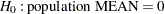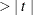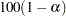### Statistics

The "Statistics" table displays all of the statistics that you request with statistic-keywords in the PROC SURVEYMEANS  statement, except DECILES, MEDIAN, Q1, Q3, and QUARTILES, which are displayed in the "Quantiles" table. If you do not specify any statistic-keywords, then by default this table displays the following information for each analysis variable: the sample size, the mean, the standard error of the mean, and the confidence limits for the mean. The "Statistics" table can contain the following information for each analysis variable, depending on which statistic-keywords you request:

• Variable name

• Variable Label

• Level, which identifies each level for categorical variables

• N, which is the number of nonmissing observations

• N Miss, which is the number of missing observations

• Minimum

• Maximum

• Range

• Number of Clusters

• Sum of Weights

• DF, which is the degrees of freedom for thetest

• Mean

• Std Error of Mean, which is the standard error of the mean

• Var of Mean, which is the variance of the mean

•Value, for testing• Pr, which is the two-sided-value for thetest

•% CL for Mean, which are two-sided confidence limits for the mean

•% Upper CL for Mean, which is a one-sided upper confidence limit for the mean

•% Lower CL for Mean, which is a one-sided lower confidence limit for the mean

• Coeff of Variation, which is the coefficient of variation for the mean

• Sum

• Std Dev, which is the standard deviation of the sum

• Var of Sum, which is the variance of the sum

•% CL for Sum, which are two-sided confidence limits for the sum

•% Upper CL for Sum, which is a one-sided upper confidence limit for the sum

•% Lower CL for Sum, which is a one-sided lower confidence limit for the Sum

• Coeff of Variation for sum, which is the coefficient of variation for the sum